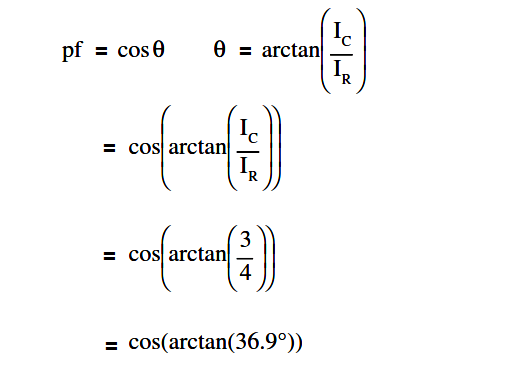# Calculate Power in Parallel RC Circuit

A 30 Ω resistance and a 40 Ω XC are in parallel with a 120V power source, as shown in Figure.Figure : Parallel R-C Circuit

Find:

1. Current, IT
2. Z
3. Power Factor, pf
4. True Power, P
5. Reactive Power, Q
6. Apparent Power, S

Solution:

##### 1.Current, ITI= 5 amps

Z = VT/IT

Z = 120/5

Z = 24 Ω

##### 3. Calculate Power factor (pf)p.f. = 0.8

##### 4. Calculate True Power, P

P = EI cos θ

P = (120)(5)(0.8)

P = 480 watts

##### 5. Calculate Reactive Power, Q

Q = EI sin θ

Q = (120)(5)(0.6)

Q = 360 VAR

S = EI

S = (120)(5)

S = 600 VA